#### 期刊菜单

Research of Preparation Boron Carbide Micro Powder by Combustion Synthesis Method
DOI: 10.12677/MEng.2020.72013, PDF, HTML, XML, 下载: 447  浏览: 619

Abstract: This paper presents a new method for the preparation of micron boron carbide powder through combustion synthesis method. In the preset work, the effect of process conditions on the product quality was systematically studied. The results show that: The adiabatic temperature of 2B2O3+C+6Mg=B4C+6MgO system is 2750 K, and the self-propagating reactions can occur spontaneously. Self-propagating reaction product mainly contains MgO, B4C and Mg3B2O6. Hydrochloric acid leaching process can effectively remove the impurity phase MgO and Mg3B2O6, thus pure B4C was obtained. The improvement of B2O3 caused the increase of Mg3B2O6 content in product, and had no significant effect on the product particle size. The improvement of Mg caused the increase of MgO content in product, and was conducive to the synthesis of B4C; when Mg excesses 5% to 15%, the product size exists a minimum value. The increase of sample making pressure can remarkably refine product granularity. Different carbon source types have significant influence on the micromorphology, particle size and purity of the product.

1. 前言

B4C具有高硬度(仅次于金刚石和立方氮化硼)、低密度(2.52 g/cm3)、高熔点(2450℃)以及高中子吸收截面(600b)等优点，广泛应用于防弹材料、耐磨和自润滑材料、切割研磨工具、防辐射材料和原子反应堆控制和屏蔽材料等；军火工业中可用作制造枪炮喷嘴    。工业生产B4C的方法是电弧炉碳热还原法，即首先矿热炉碳热还原得到碳化硼结晶块，经破磨，湿法酸洗净化得到碳化硼粉体，该工艺存在粒度大(微观晶粒尺寸10~20 µm，微米级碳化硼超微粉 < 2.0 µm)、杂质含量高、烧结活性差等缺馅，且破碎磨细过程    造成二次污染，导致产品纯度下降。激光诱导化学气相沉积法是以含有碳源及硼源的气体(BCl3、B2H6、CHCl3、CH4等)为原料，在激光辐射下混合气体迅速升温反应生成B4C纳米颗粒，再经过处理得到具有纯净的纳米B4C粉 。溶胶–凝胶法也是制备B4C超细粉的一种新方法，Sinha用硼酸/柠檬酸凝胶前驱体在1000℃~1450℃保温2 h，得到平均粒径为2.25 µm的B4C微粉 。目前，B4C超细微粉制备仍旧是研究热点和难点，其制备技术只有少数几个国家掌握。燃烧合成法作为一种超细粉体的方法，制备的粉体具有高活性等优点  - 。本文系统研究了工艺条件对燃烧合成制备B4C粉体品质的影响。

2. 实验

2.1. 实验步骤

2.2. 检测分析

3. 结果与讨论

3.1. B2O3-Mg-C反应的热力学分析

$\Delta H=\Delta {H}_{298}^{\theta }+{\int }_{298}^{{T}_{ad}}\Delta {c}_{p}\text{d}T$

$\Delta {H}_{298}^{\theta }$ 为在298 K时反应的标准摩尔反应焓变，由反应物与产物的标准摩尔生成焓相减得，单位是kJ∙mol−1

$\Delta {c}_{p}$ 为产物在温度T下的热容，单位是J∙K−1∙mol−1

${c}_{p}$ 可近似的用以下公式计算：

${c}_{p}={A}_{1}+{A}_{2}×{10}^{-3}T+{A}_{3}×{10}^{5}{T}^{-2}+{A}_{4}×{10}^{-6}{T}^{2}+{A}_{5}×{10}^{8}{T}^{-3}$

A1，A2，A3，A4，A5可由无机热力学数据手册査得。

$\Delta {H}_{298}^{\theta }=-1138.130\text{\hspace{0.17em}}\text{kJ}/\text{mol}$

$-\Delta {H}_{298}^{\theta }={\int }_{298}^{{T}_{ad}}\Delta {c}_{p}\text{d}T+\Delta {H}_{m}+{\int }_{{T}_{m}}^{{T}_{ad}}\Delta {C}_{p液}\text{d}T$

${\text{B}}_{\text{2}}{\text{O}}_{\text{3}}+\text{3Mg}=\text{2B}+\text{3MgO}$ (1)

$\text{4B}+\text{C}={\text{B}}_{\text{4}}\text{C}$ (2)

$\text{3MgO}+{\text{B}}_{\text{2}}{\text{O}}_{\text{3}}={\text{Mg}}_{\text{3}}{\text{B}}_{\text{2}}{\text{O}}_{\text{6}}$ (3)

${\text{2B}}_{\text{2}}{\text{O}}_{\text{3}}+\text{6Mg}+\text{C}={\text{B}}_{\text{4}}\text{C}+\text{6MgO}$ (4)

$\text{2Mg}+\text{3C}={\text{Mg}}_{\text{2}}{\text{C}}_{\text{3}}$ (5)

${\text{2B}}_{\text{2}}{\text{O}}_{3}+\text{3C}=\text{4B}+{\text{3CO}}_{2}$ (6)

${\text{2B}}_{\text{2}}{\text{O}}_{\text{3}}+\text{7C}={\text{B}}_{\text{4}}\text{C}+\text{6CO}$ (7)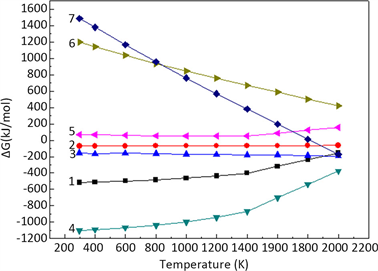Figure 1. The Gibbs free energy changes of the SHS reaction

3.2. B2O3配料量对燃烧合成反应的影响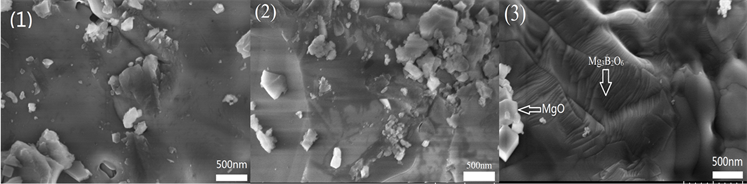Figure 2. SEM photographs of combusted products at different B2O3 contents; (1) Excess 5% B2O3; (2) Excess 10% B2O3; (3) Stoichiometric ratio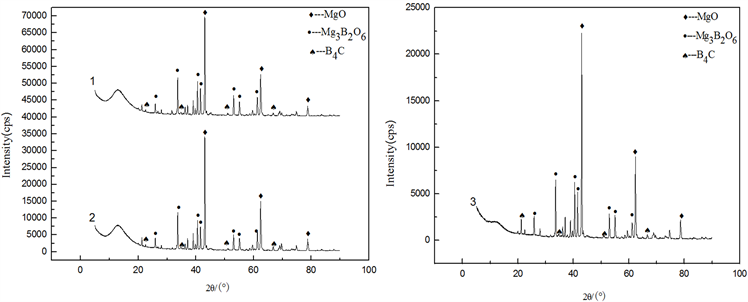Figure 3. XRD patterns of combusted products at different B2O3 contents; (1) Excess 5% B2O3; (2) Excess 10% B2O3; (3) Stoichiometric ratio

${\text{2B}}_{\text{2}}{\text{O}}_{\text{3}}+\text{6Mg}+\text{C}={\text{B}}_{\text{4}}\text{C}+\text{6MgO}$ (8)

$\text{3MgO}+{\text{B}}_{\text{2}}{\text{O}}_{\text{3}}={\text{Mg}}_{\text{3}}{\text{B}}_{\text{2}}{\text{O}}_{\text{6}}$ (9)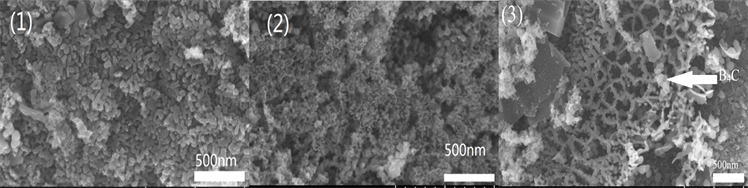Figure 4. SEM photographs of leached products at different B2O3 contents; (1) Excess 5% B2O3; (2) Excess 10% B2O3; (3) Stoichiometric ratio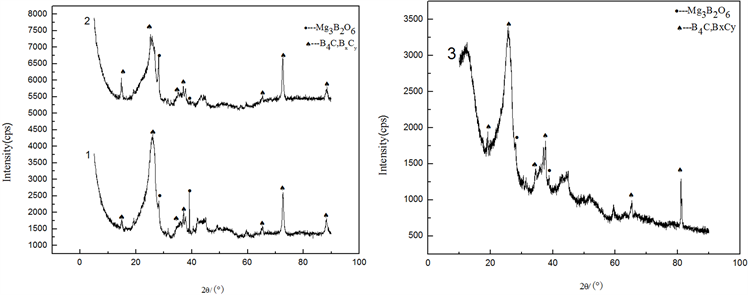Figure 5. XRD patterns of leached products at different B2O3 contents; (1) Excess 5% B2O3; (2) Excess 10% B2O3; (3) Stoichiometric ratio

$\text{MgO}+\text{2HCl}={\text{MgCl}}_{\text{2}}+{\text{H}}_{\text{2}}\text{O}$ (10)

${\text{Mg}}_{\text{3}}{\text{B}}_{\text{2}}{\text{O}}_{\text{6}}+\text{6HCl}={\text{3MgCl}}_{\text{2}}+{\text{2H}}_{\text{3}}{\text{B}}_{\text{2}}{\text{O}}_{\text{3}}$ (11)Table 1. Mg and C content of leached products at different B2O3 contents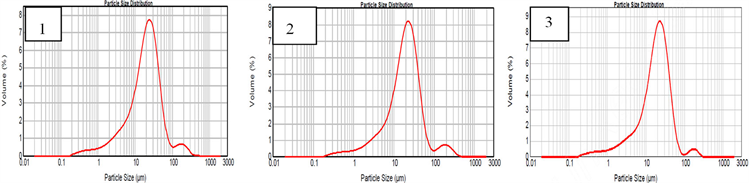Figure 6. Particle size distribution curve of at different B2O3 contents; (1) Excess 5% B2O3; (2) Excess 10% B2O3; (3) Stoichiometric ratio

3.3. 制样压力对燃烧合成反应的影响Figure 7. XRD patterns of combusted products at different sample preparation pressures; (1) 20 MPa; (2) 40 MPa; (3) 60 MPa; (4) 80 MPa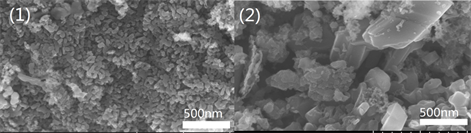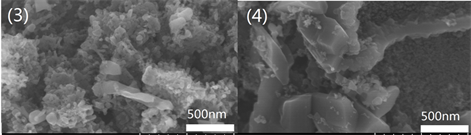Figure 8. SEM photographs of combusted products at different sample preparation pressures; (1) 20 MPa; (2) 40 MPa; (3) 60 MPa; (4) 80 MPa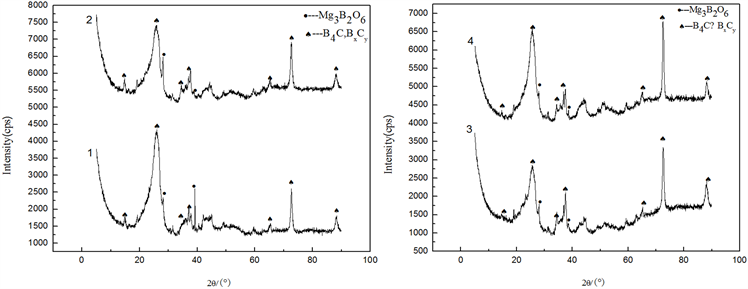Figure 9. XRD patterns of leached products at different sample preparation pressures; (1) 20 MPa; (2) 40 MPa; (3) 60 MPa; (4) 80 MPaTable 2. Mg and C content of leached products at different sample preparation pressures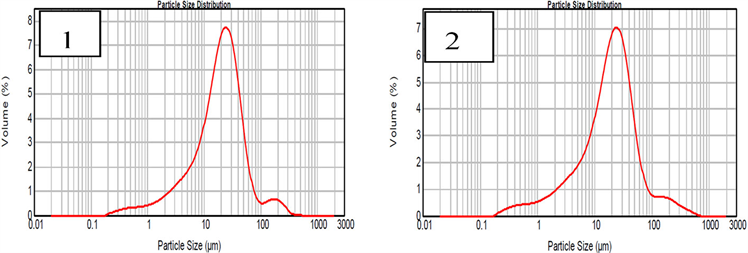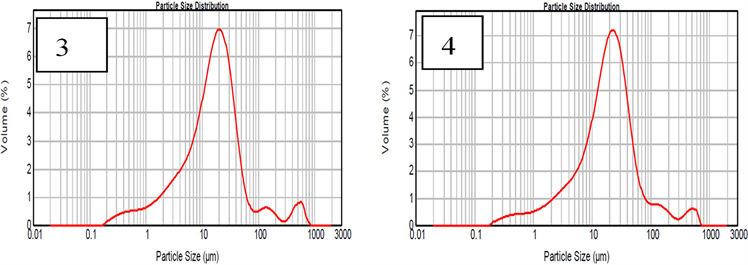Figure 10. Particle size distribution curve at different sample preparation pressures (1) 20 MPa, (2) 40 MPa, (3) 60 MPa, (4) 80 MPa

4. 结论

1) ${\text{2B}}_{\text{2}}{\text{O}}_{\text{3}}+\text{C}+\text{6Mg}={\text{B}}_{\text{4}}\text{C}+\text{6MgO}$ 体系的绝热温度为2750 K，大于1800 K的热力学判据，说明反应可以自我维持发生。燃烧产物主要由B4C、MgO以及少量Mg3B2O6相组成；采用盐酸浸出可有效除去其中的MgO和Mg3B2O6相，得到B4C粉体。

2) 随氧化硼配料量增加可降低产品中的Mg含量，但对产物粒度无明显影响；坯样压力为60 MPa时，浸出产物中C含量为23.4%，与B4C中C理论含量21.73%接近，此时平均粒径为16.276 µm。

  章晓波, 刘宁. 碳化硼材料的性能, 制备与应用[J]. 硬质合金, 2006(2): 120-125.  Kitamura, J., Usuba, S., Kakudate, Y., et al. (2003) Boron Carbide Coating by Electromagnetically Accelerated Plasma Spraying. Journal of Thermal Spray Technology, 12, 70-76. https://doi.org/10.1361/105996303770348519  吴芳, 吕军. 碳化硼陶瓷的制备及应用[J]. 五邑大学学报(自然科学版), 2002(1): 45-49.  刘维良. 先进陶瓷工艺学[M]. 武汉: 武汉理工大学出版社, 2004.  贾宝瑞, 秦明礼, 李慧, 等. 碳化硼粉末制备方法的研究进展[J], 材料导报, 2010(9): 32-34.  于国强, 刘维良, 欧阳瑞丰, 等. 碳热还原法制备碳化硼粉末的工艺研究[J]. 中国陶瓷, 2012, 48(6): 58-59.  Atasoy, A. (2010) The Aluminothermic Reduction of Boric Acid. International Journal of Refractory Metals & Hard Materials, 28, 616-622. https://doi.org/10.1016/j.ijrmhm.2010.06.001  谢洪勇. 机械化学法制备碳化硼及其晶体结构的研究[J]. 上海第二工业大学学报, 2006, 23(2): 122-126.  Li, S., Zeng, B., Feng, Z., et al. (2010) Effects of Heat Treatment on the Microstructure of Amorphous Boron Carbide Coating Deposited on Graphite Substrates by Chemical Vapor Deposition. Thin Solid Films, 519, 251-258. https://doi.org/10.1016/j.tsf.2010.08.099  李蓓, 简敏, 王美玲, 等. 碳化硼粉末的制备方法[J]. 核动力工程, 2012(S2): 110-113.  葛禹锡, 黄锋, 倪红军, 等. 自蔓延高温合成法制备粉体的研究进展[J]. 热加工工艺, 2012, 41(12): 75-78.  张化宇, 韩杰才, 王华彬, 等. 气压对自蔓延高温还原合成B4C的影响[J]. 中国有色金属学报, 1999(S1): 190-194.  张化宇, 韩杰才, 赫晓东, 等. 自蔓延高温合成MgO-B4C微观组织研究[J]. 宇航材料工艺, 2000, 30(2): 25-28.  Jiang, G., Xu, J., Zhuang, H., et al. (2011) Fabrication of B4C from Na2B4O7+Mg+C by SHS Method. Ceramics International, 37, 1689-1691. https://doi.org/10.1016/j.ceramint.2010.10.007  Jiang, G., Xu, J., Zhuang, H., et al. (2009) Combustion of Na2B4O7+Mg+C to Synthesis B4C Powders. Journal of Nuclear Materials, 393, 487-491. https://doi.org/10.1016/j.jnucmat.2009.07.008